# How to Calculate and Solve for Mutually Exclusive | Probability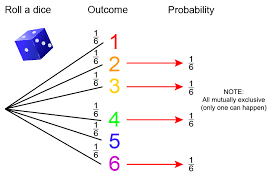The image above represents mutually exclusive.

To compute for mutually exclusive, four essential parameters are needed and these are xA, NA, xB and NB.

The formula for calculating mutually exclusive:

P(A or B) = P(A) + P(B)

Where;

P(A or B) = Mutually Exclusive
P(A) = xANA
P(B) = xBNB

Let’s solve an example;
Find the mutually exclusive when the xA is 12, NA is 14, xB is 9 and NB is 17.

This implies that;

xA = 12
NA = 14
xB = 9
NB = 17

P(A or B) = P(A) + P(B)
P(A or B) = xANA + xBNB
P(A or B) = 1214 + 917
P(A or B) = 12(17) + 9(14)(14)(17)
P(A or B) = 204 + 126238
P(A or B) = 330238
Dividing the numerator and denominator by 2
P(A or B) = 165119
P(A or B) = 1.386

Therefore, the mutually exclusive is 1.386.

Nickzom Calculator – The Calculator Encyclopedia is capable of calculating the mutually exclusive.

To get the answer and workings of the mutually exclusive using the Nickzom Calculator – The Calculator Encyclopedia. First, you need to obtain the app.

You can get this app via any of these means:

To get access to the professional version via web, you need to register and subscribe for NGN 1,500 per annum to have utter access to all functionalities.
You can also try the demo version via https://www.nickzom.org/calculator

Apple (Paid) – https://itunes.apple.com/us/app/nickzom-calculator/id1331162702?mt=8
Once, you have obtained the calculator encyclopedia app, proceed to the Calculator Map, then click on Probability under Mathematics.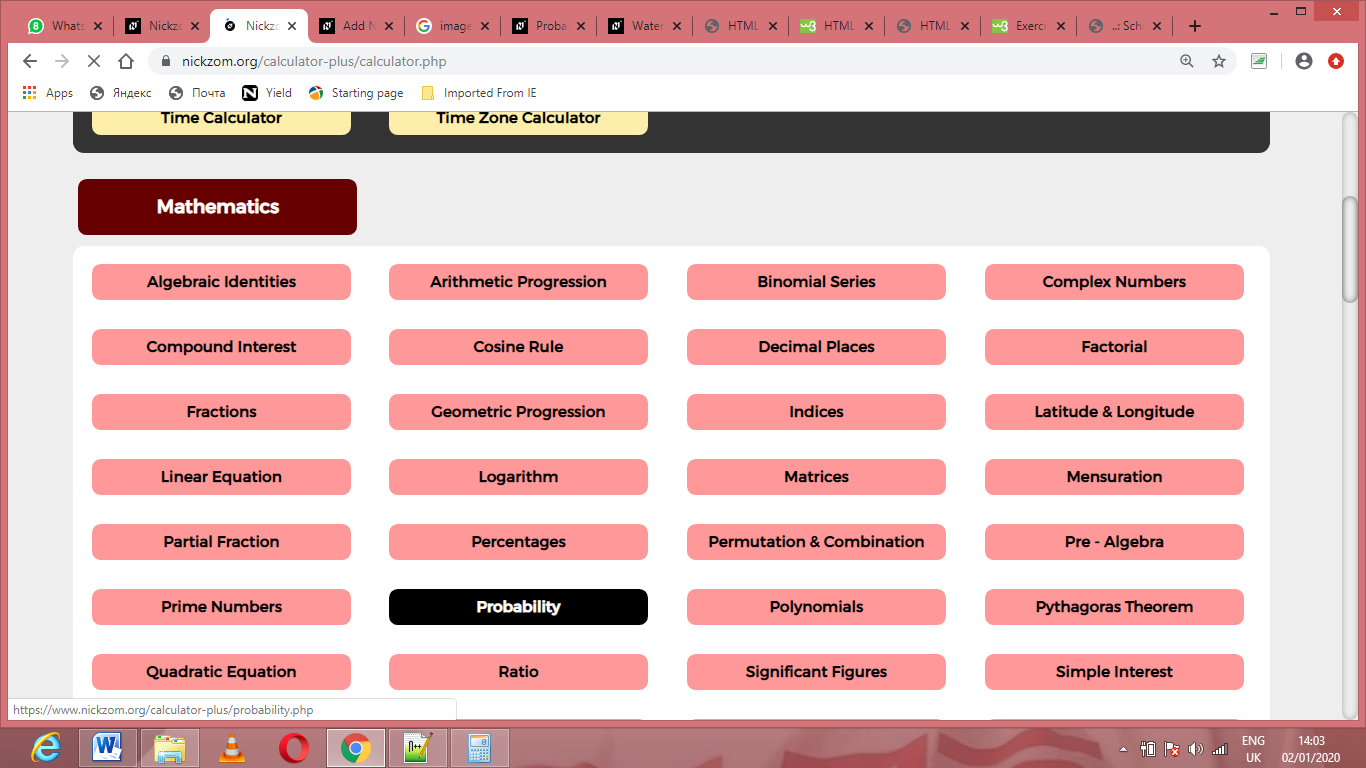Now, Click on Mutually Exclusive under Probability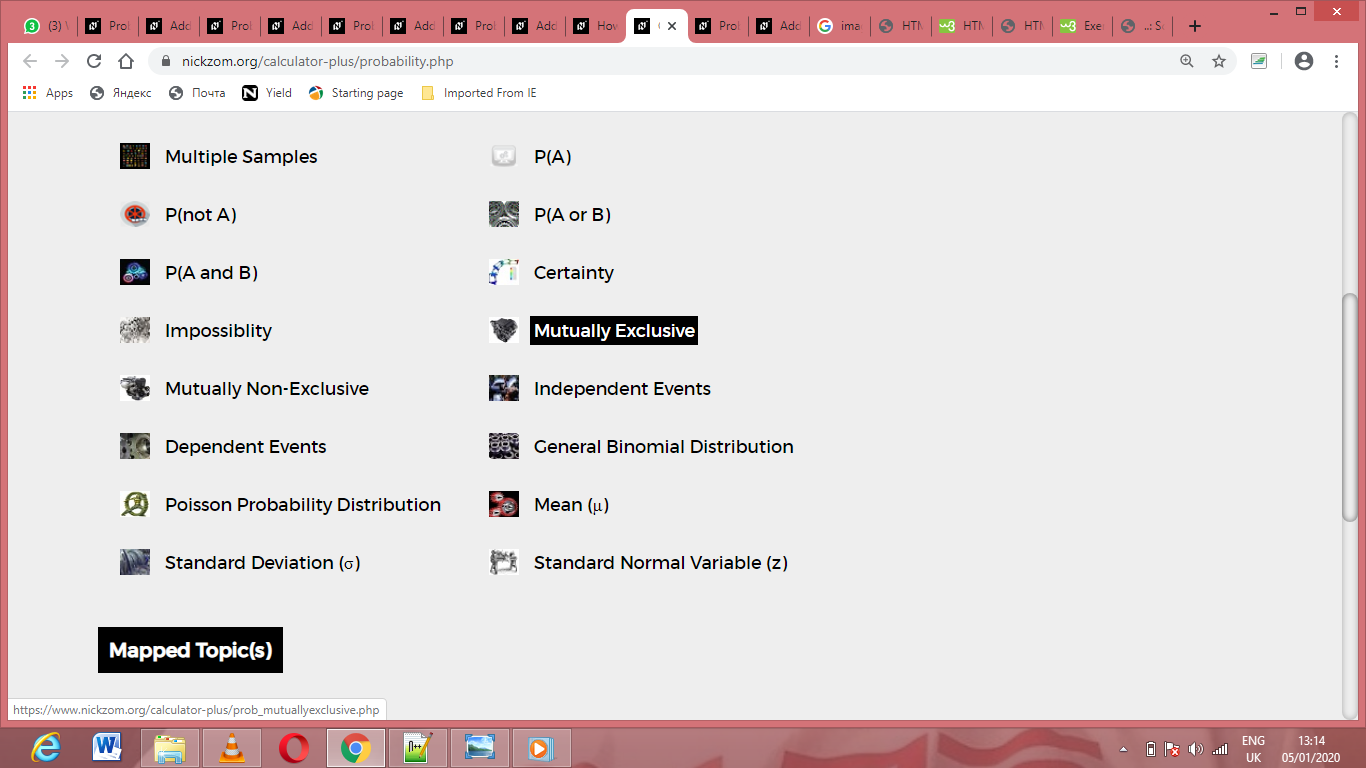The screenshot below displays the page or activity to enter your values, to get the answer for the mutually exclusive according to the respective parameters which are the xA, NA, xB and NB.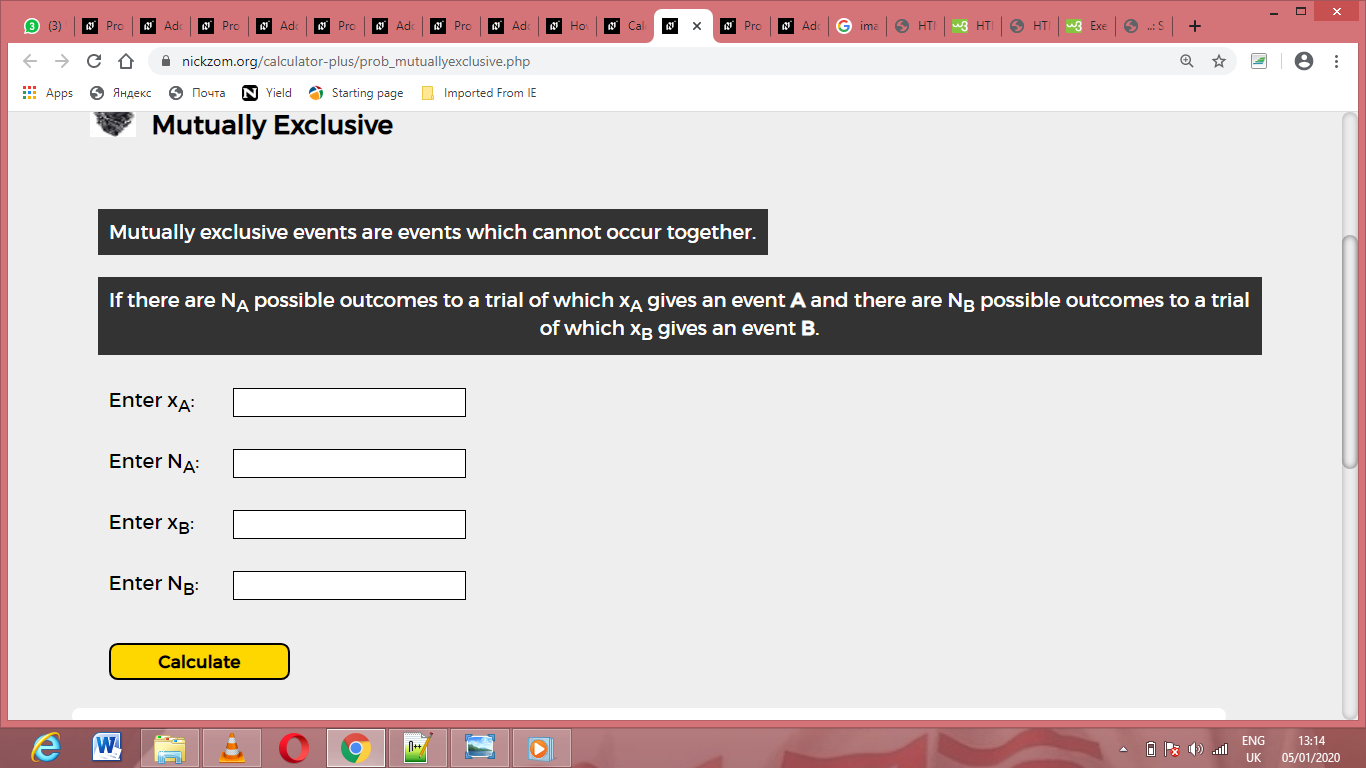Now, enter the values appropriately and accordingly for the parameters as required by the xA is 12, NA is 14, xB is 9 and NB is 17.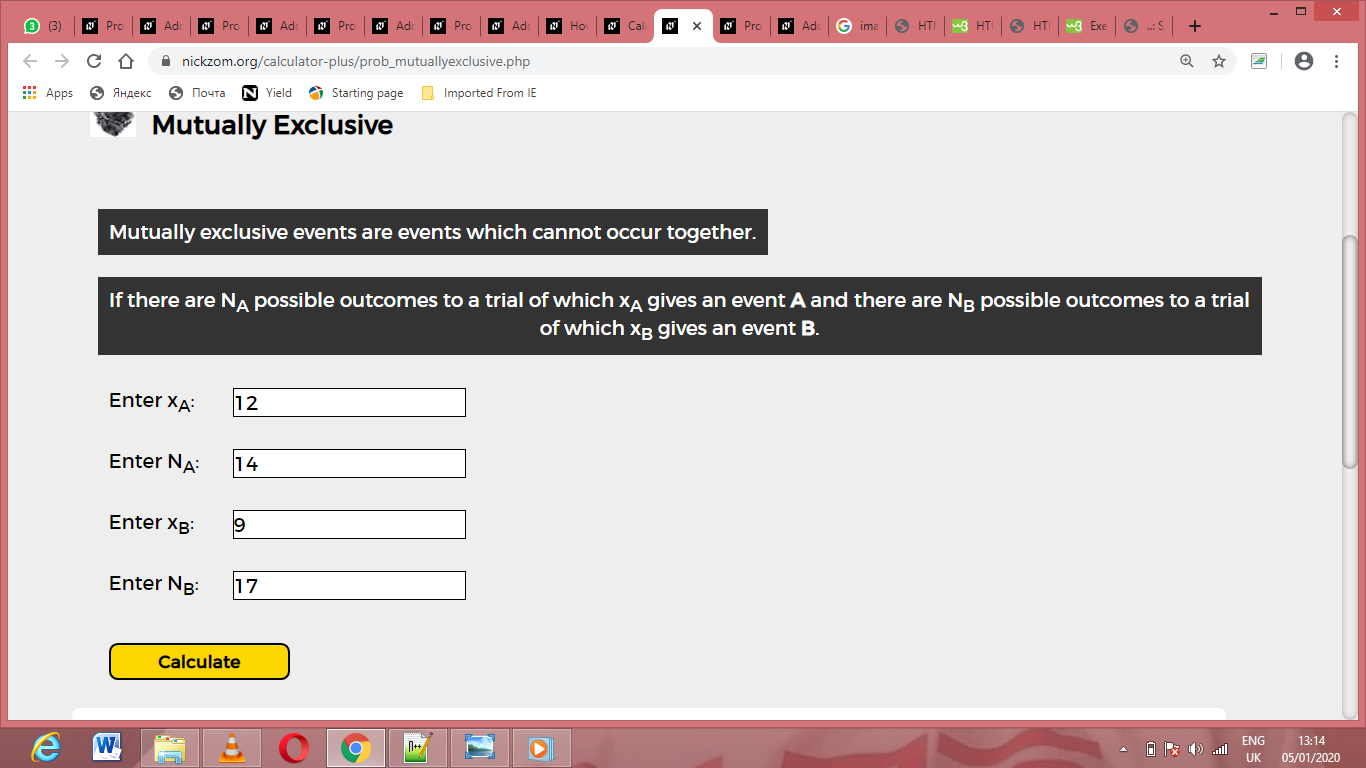Finally, Click on Calculate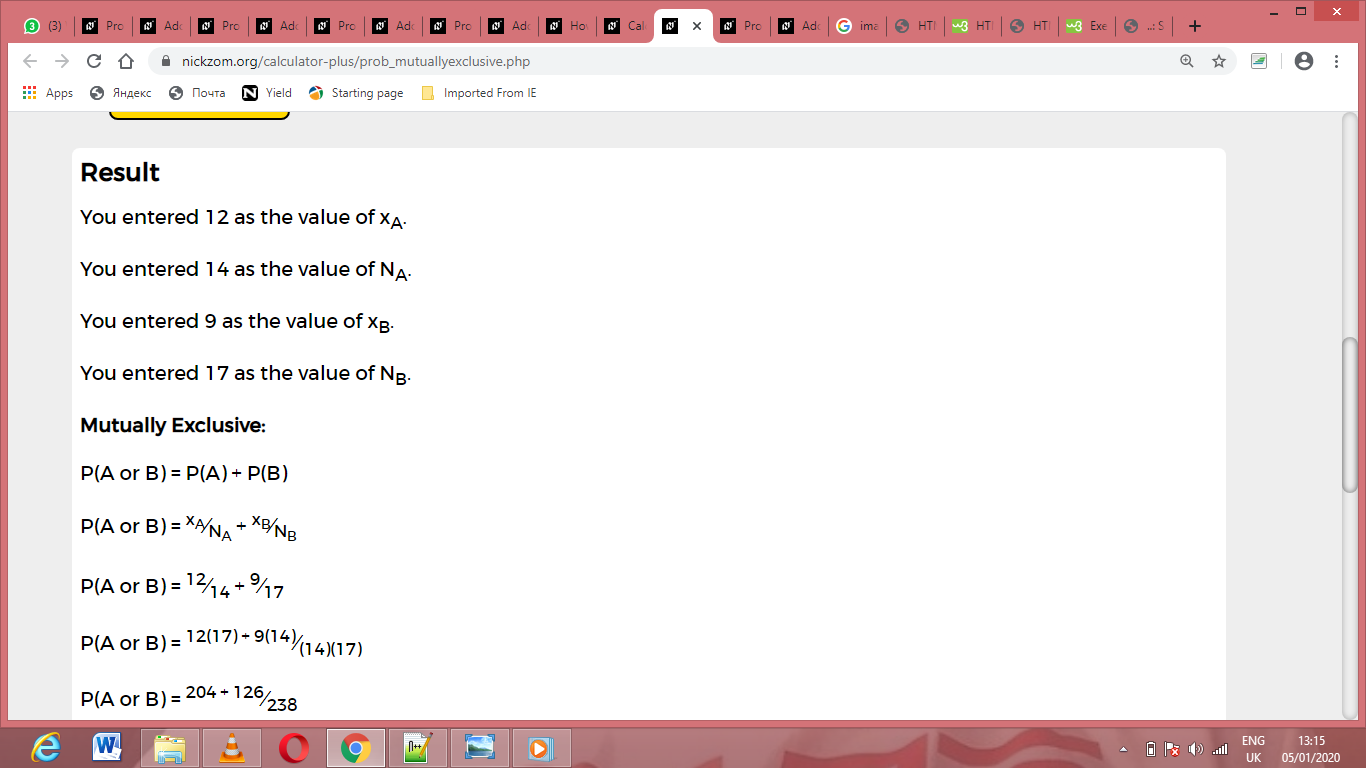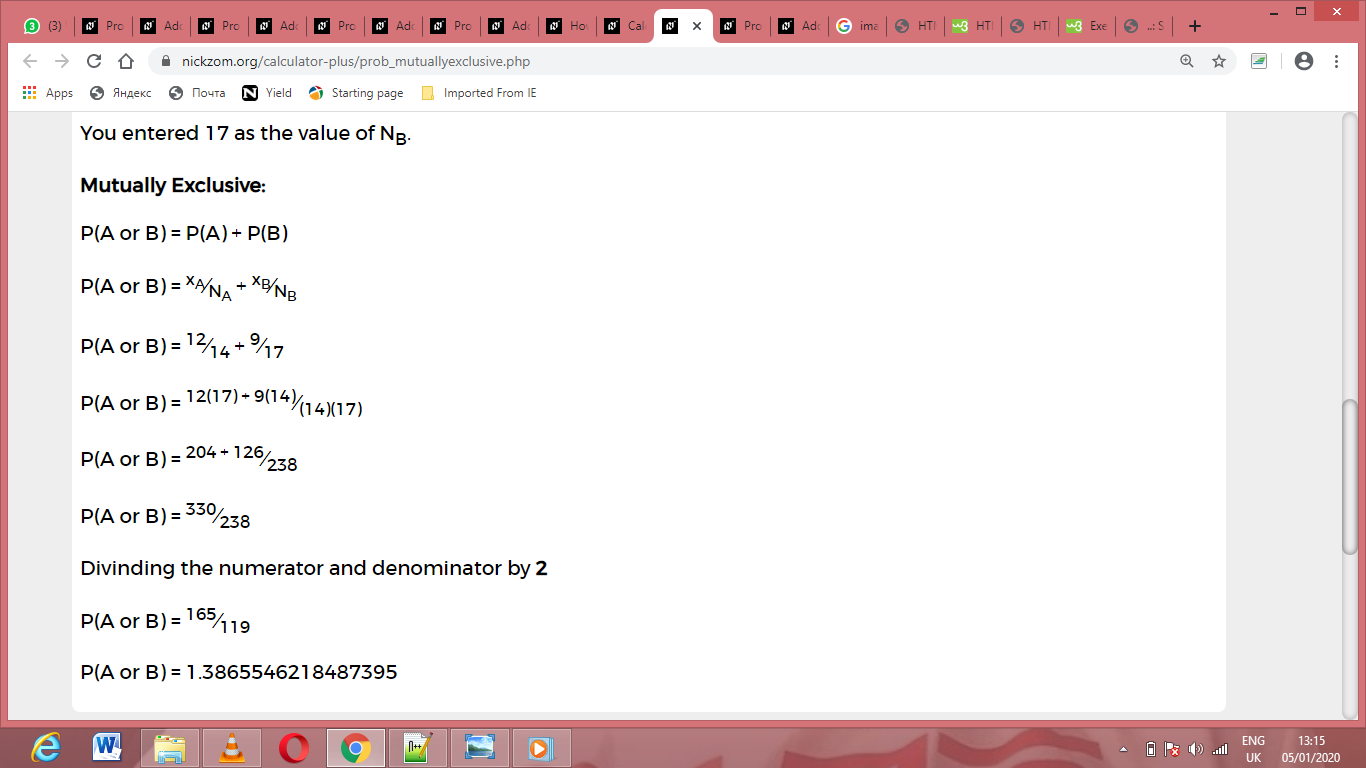As you can see from the screenshot above, Nickzom Calculator– The Calculator Encyclopedia solves for the mutually exclusive and presents the formula, workings and steps too.